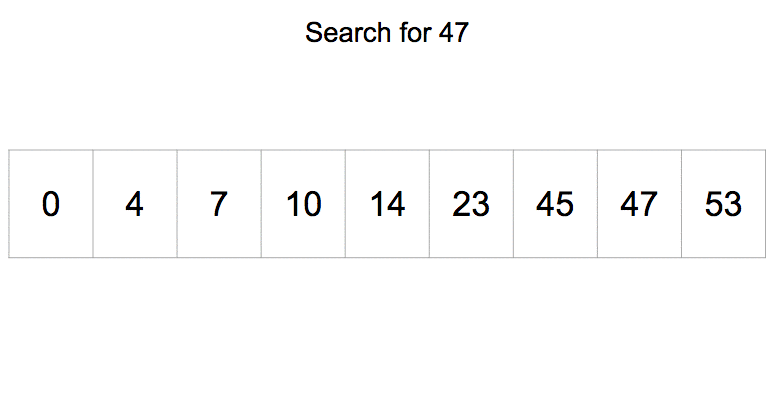# Binary Search in ABAP

Binary Search in ABAP is an important topic in terms of interview question. In general, ABAP provides keyword “SEARCH” for searching purpose and that is widely used everywhere. In this article, we will learn: What is a Binary Search and how to implement it in our ABAP programs.

What is a Binary Search?

A Binary Search is a search method in which a particular value is searched from an array (in case of ABAP, Internal Table) of values via a procedure as listed below:

1. The whole array is firstly sorted.
2. Then the searched value is compared with the middle value.
3. If it is equal, then output is shown with index/position of value.
4. If it is less than the middle value, then values after middle value is discarded and if it is greater than the middle value then the values before middle value is discarded.
5. Repeat step 4 till we achieve step 3. If it is not achieved till end then “Not Found”  message is printed on the screen. The process is shown below using image (click on the image) and program:Binary Search in ABAP:

```DATA : wa_data type int4,
ITAB_data type TABLE OF int4,
last_num type int1, " Index of Last Number
first_num type int1 value 1, " Index of First number
flag type int1 value 0,
pos_length type int1.
SELECTION-SCREEN BEGIN OF SCREEN 0100 . "Where we create a Screen 0100
SELECT-OPTIONS: s_data FOR wa_data. "Seclect-Option for multiple Input
PARAMETERS: p_input type int4.
SELECTION-SCREEN END OF SCREEN 0100.
DATA: wa_sdata LIKE LINE OF s_data.
START-OF-SELECTION.
CALL SELECTION-SCREEN 0100.
LOOP AT s_data INTO wa_sdata.
APPEND wa_sdata-low TO ITAB_data.
ENDLOOP.
DESCRIBE TABLE ITAB_data LINES last_num." Here we get total number of record and currently it the index of last number
Sort ITAB_data.
pos_length = ( last_num ) / 2 .
while ( first_num <= last_num ).
READ TABLE ITAB_data INTO wa_data INDEX pos_length.
IF ( p_input = wa_data ) .
flag = 1.
Exit.
ELSEIF ( p_input < wa_data ).
last_num =  pos_length - 1.
pos_length = ( first_num + last_num ) / 2 .
ELSE.
first_num = pos_length + 1.
pos_length = ( first_num + last_num ) / 2 .
ENDIF.
ENDWHILE.
IF ( Flag = 1 ).
Write: 'Hurray! Found  your input @ position', pos_length.
ELSE.
ENDIF.

```

Explanation

In the program above, initially we have defined data types: One internal tables [to store input array of data], one work area [To be used by the above mentioned internal tabls],  one variable [to save current line/index], one variable [to store current position], one variable to be used as a flag, two variable [to store first and last variable of internal table].

We have then created a selection screen to take input and save it in an internal table. Now we will be using DESCRIBE, we will get the total number of records in that internal table. Then we will sort the internal table using “SORT” statement.

Then we use a loop and use the algorithm steps mentioned above and set a flag as 1, if data is found. And in last, print either “Found” with position of the data or “Not Found”.